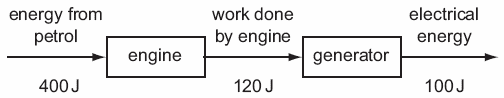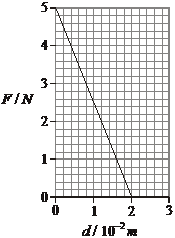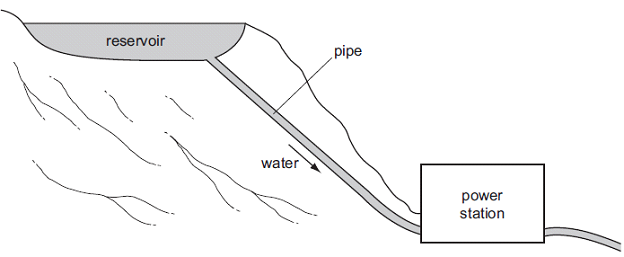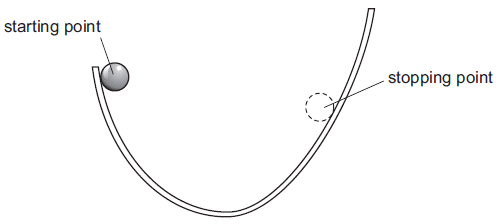1. Energy from petrol is used to operate an engine. The engine drives a generator, which produces electrical energy.What is the overall efficiency of the process?

2. The graph below shows the variation with displacement d of the force F applied by a spring on a cart.The work done by the force in moving the cart through a distance of 2 cm is

A.      10 × 10–2J.

B.       7 × 10–2J.

C.      5 × 10–2J.

D.         2.5 × 10–2J.

3.An engine takes in an amount E of thermal energy and, as a result, does an amount W of useful work. An amount H of thermal energy is ejected. The law of conservation of energy and the efficiency of the engine are given by which of the following?

 Law of conservation of energy Efficiency A. E = W + H W B. E = W + H W/E C. E + H = W W/H D. E + H = W W/(E-H)

4. A machine lifts an object of weight 1.5 × 103 N to a height of 10 m. The machine has an overall efficiency of 20%. The work done by the machine in raising the object is

A.      3.0 × 103 J.

B.       1.2 × 104 J.

C.      1.8 × 104 J.

D.      7.5 × 104 J.

5. An electric train develops a power of 1.0 MW when travelling at a constant speed of 50 ms–1. The net resistive force acting on the train is

6. Which one of the following is a true statement about energy?

7 . The diagram shows a hydroelectric system.What are the main energy changes taking place?

8. An electric motor, with an input power of 250 W, produces 200 W of mechanical power. The efficiency of the motor isThe ball is released. It rolls down one side of the track and part of the way up the other side. It then stops, before rolling back down again. The height of the stopping point is less than that of the starting point.

What is the sequence of energy changes between starting and stopping for the first time?

10. It is easier to roll a stone up a sloping road than to lift it vertical upwards because

Question 1 of 10